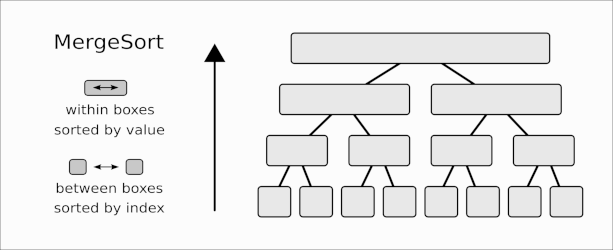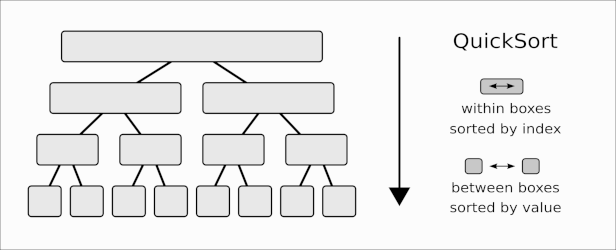# A dual view of sorting algorithms

Mergesort and Quicksort are two well-known sorting algorithms, familiar to both researchers and practitioners. Both are typically presented as examples of divide and conquer. Other than this fact, they are usually not seen to be very closely related. Let’s look at Mergesort and Quicksort in a somewhat unusual way that will make them appear as two sides of the same coin.

Suppose we want to sort a list of items $$a_1, a_2, \dots, a_n$$.

In Mergesort, we split the list into two parts: $$L = a_1, \dots, a_{n/2}$$, and $$R = a_{n/2+1}, \dots, a_n$$. We recursively sort $$L$$ and $$R$$, then merge the two sorted lists to obtain the final, sorted output.

How do we sort $$L$$ and $$R$$ recursively? We split them further into equal parts (let’s assume for simplicity that $$n$$ is a power of two, so this always works), until parts consist of single elements, which are already trivially sorted.

So, at the bottom of the recursion tree, we have $$n$$ singleton-lists, consisting of $$a_1, a_2, \dots, a_n$$. Assuming that the splitting has happened “in place”, these items are still stored in their original order, “sorted by their index” $$1,2,\dots,n$$.

See the following illustration.The bottom-most line contains the elements in their original order, one in each “box”. As we come out of the recursion, we merge neighboring “boxes” in pairs, putting their elements in the correct order. So within each box, elements are sorted “by value“.

Observe that neighboring boxes in the same line are sorted “by index“, in the sense that their ordering preserves the original order: indices in any box are smaller than indices in the next box to the right. For example in the second line from the bottom, the first box will contain $$(a_1, a_2)$$ or $$(a_2, a_1)$$, the next box will contain $$(a_3, a_4)$$ or $$(a_4, a_3)$$, etc. the point being that 1 and 2 are both smaller than 3 and 4.

This is Mergesort.

Can we reverse the arrow of time and go from the top towards the bottom? Well, that would be strange, because at the top we have a single box, so this would mean that we start with a single list that is already sorted, which wouldn’t make sense.

But what if by “sorted” we meant “sorted by index“, meaning that the entries are stored as $$(a_1, a_2, \dots, a_n)$$. There is of course no harm in assuming this, as this is indeed how the input is presented. So let’s update the figure.

Instead of bottom-to-top, let’s go top-to-bottom. Let’s also swap “by value” and “by index” throughout.

Now we go from a single box, sorted by index (meaning an unsorted input list) to a list of boxes sorted among them by value (meaning a sorted output list in the bottom). So conceptually, this looks very much like a valid sorting algorithm. What is it? Does it have a name?Yes, it is Quicksort.

Let’s think it through again: we start with a list (sorted “by index”), and we produce from it two lists, sorted between them “by value”. This means that all entries in one are smaller than all entries in the other (this is essentially the partitioning step of Quicksort: we split the elements into those smaller, and those greater or equal than a pivot).

What does it mean that “within a box” the entries are now sorted “by index”? This just means that on both sides of the partitioning we preserve the original ordering of entries. This is not true in every implementation of Quicksort, but it is a technicality we can certainly enforce if we want to. Observe that we assumed that Quicksort splits the list in two equal parts, something we can achieve if we use the median as pivot (in practice of course a random pivot works just fine and is usually faster).

So there you have it. Quicksort and Mergesort are “duals”: you get one from the other by running time backwards, and swapping “by value” and “by index” in the invariants they maintain (or alternatively, swapping “between” and “within”). Yet another way to look at it is that Quicksort does a similar thing as Mergesort, but on the inverse permutation as input.

Surprising? Obvious? Is this view of practical use?